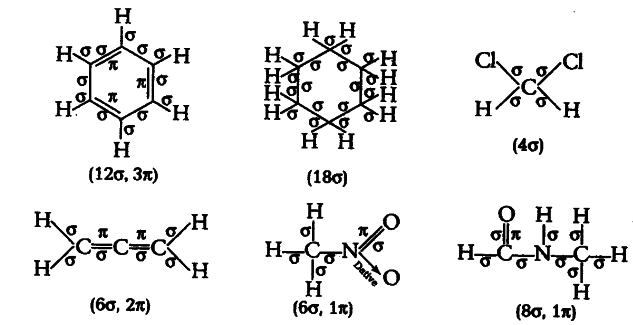# Indicate the σ- and $\pi$-bonds

Indicate the σ- and $\pi$-bonds in the following molecules.
$C_{6}H_{6}$, $C_{6}H_{12}$, $CH_{2}Cl_{2}$,
${ CH }{ 2 }$=C=
${ CH } { 2 }$,
$CH_{3}NO_{2}$,
${ HCONHCH }_{ 3 }$